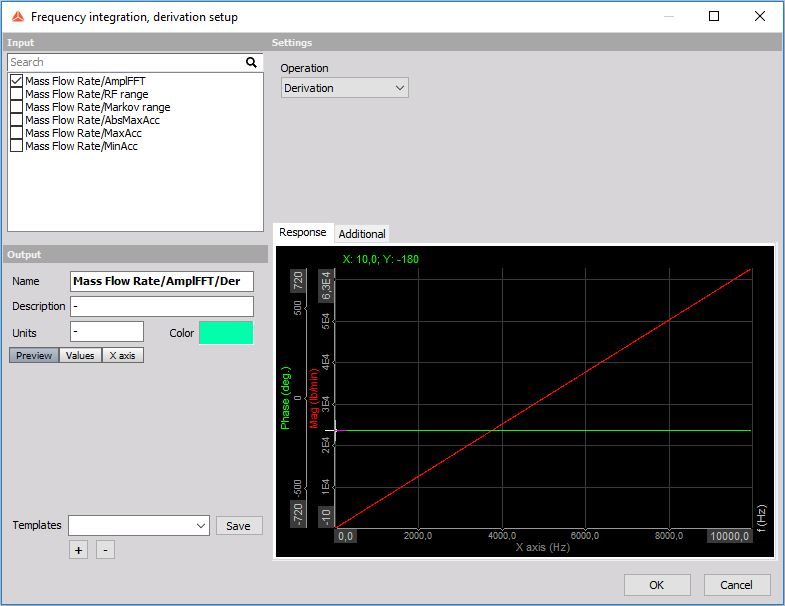# Frequency integration, derivation

When opening a new Frequency integration, derivation math function the following setup window will appear:Under settings we can select the type of operation:

Integral functions

• Integration (used for functions where the carrier of information is the DC value (the offset) or the dynamic part of the signal.)

• Double integration (used for dynamic signals)

Derivative functions